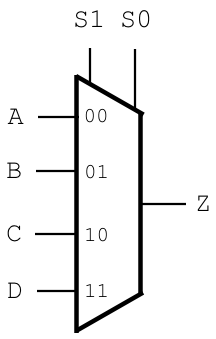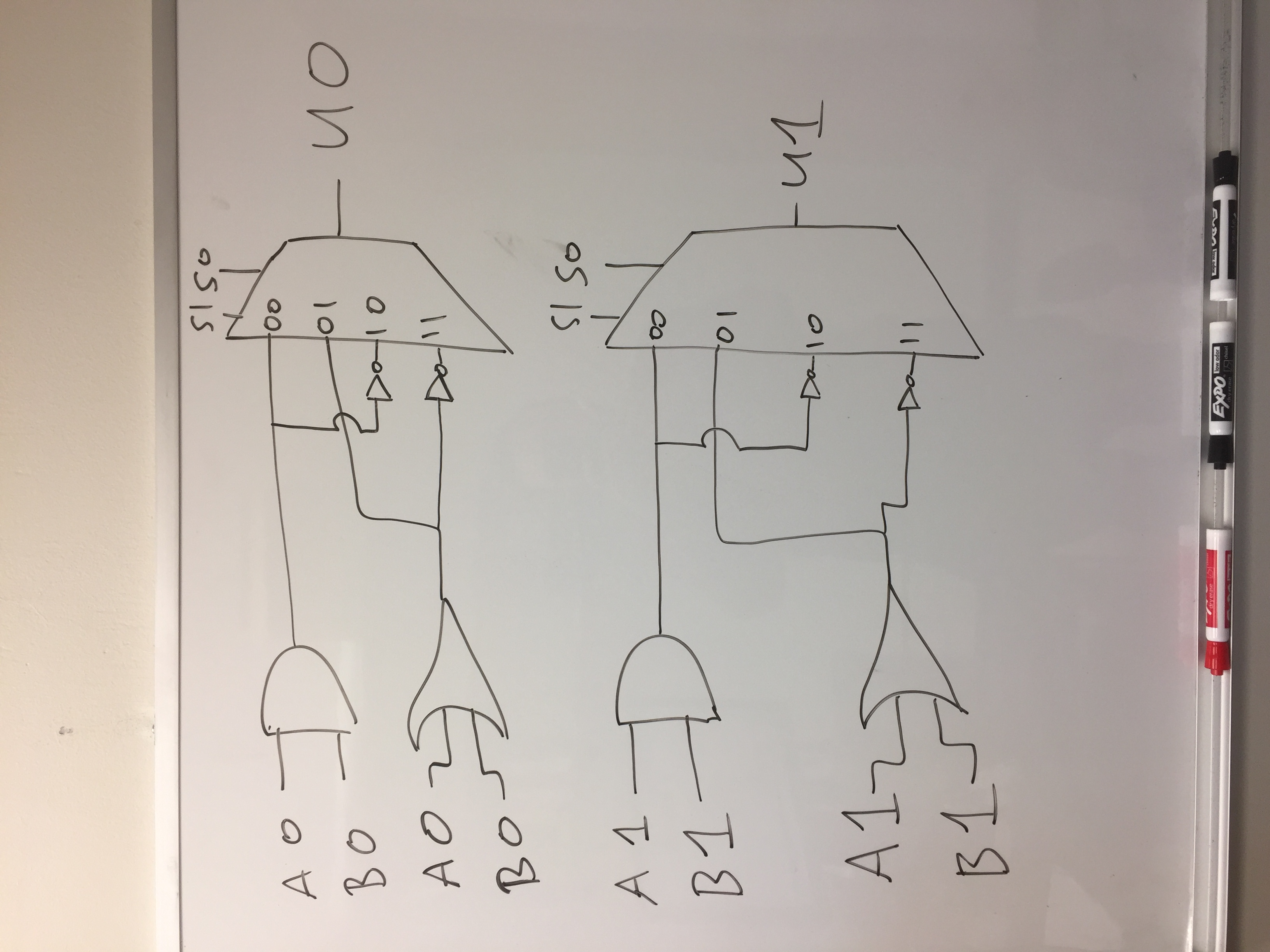# Final Review Questions (with Answers)

The final exam will be synchronous during class and lab time on Tuesday, August 23, 2022. This will run from 10 AM to 11:20 PM. You are not required to join the Zoom for the class during the time, but the exam will be available only during this time, via Canvas.

# Comprehensive Exam and Review

The final exam will be comprehensive, but with a bias towards content taught since the last exam. It is recommended to review the following:

• Prior exams
• The exam reviews for the prior exams

# Questions

1. What component is shown below?`An `OR` gate.`
2. What component is shown below?`An `AND` gate.`
3. What component is shown below?`A `NOT` gate.`
4. Draw the circuit corresponding to the following sum-of-products equation, where `!A` refers to the negation of variable `A`, and so on:
```R = !A!B + AB
```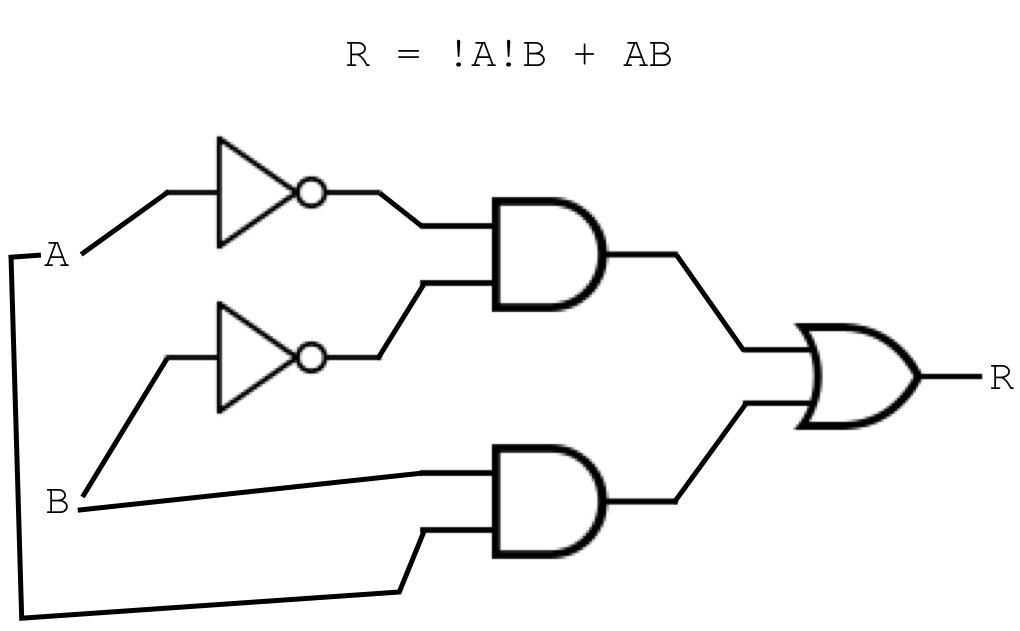5. Consider the following sum-of-products equation:
```R = !ABC + ABC + A!B!C
```

Using the above equation, do the following:

• Write it as a truth table: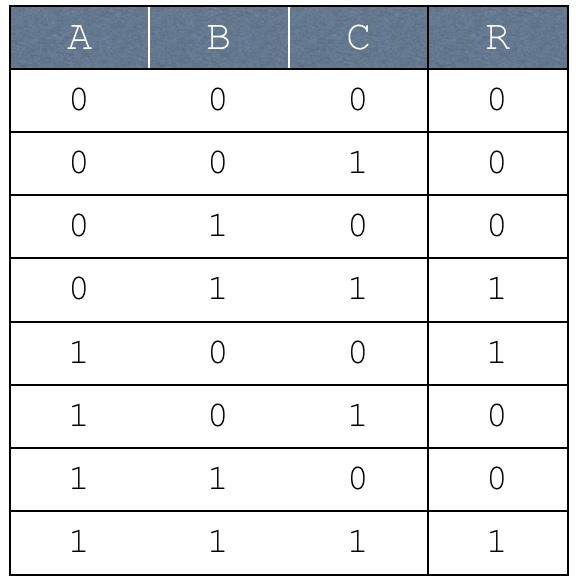• Simplify it using boolean algebra: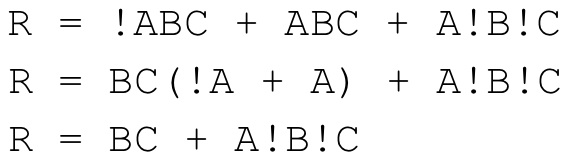• Simplify it using a Karnaugh map: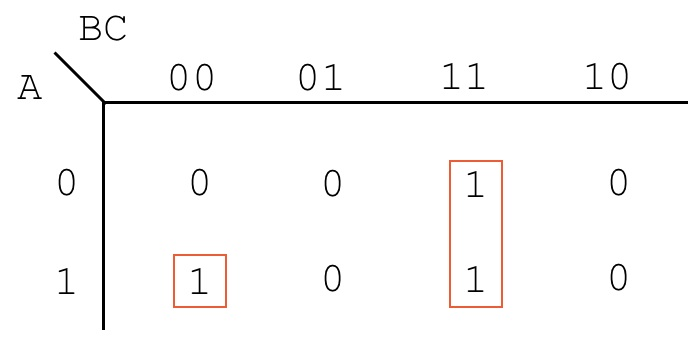`R = A!B!C + BC`
6. Consider the truth table augmented with don't cares, shown below:
A B C D U
00001
0001X
00100
00111
0100X
01011
01100
0111X
10001
10010
1010X
10110
11001
11010
1110X
11110

Using the above truth table, write out the following:

1. The unoptimized sum-of-products equation, skipping over don't cares
```              U = !A!B!C!D + !A!BCD + !AB!CD + A!B!C!D + AB!C!D
```
2. A Karnaugh map, along with boxes which exploit don't cares where appropriate.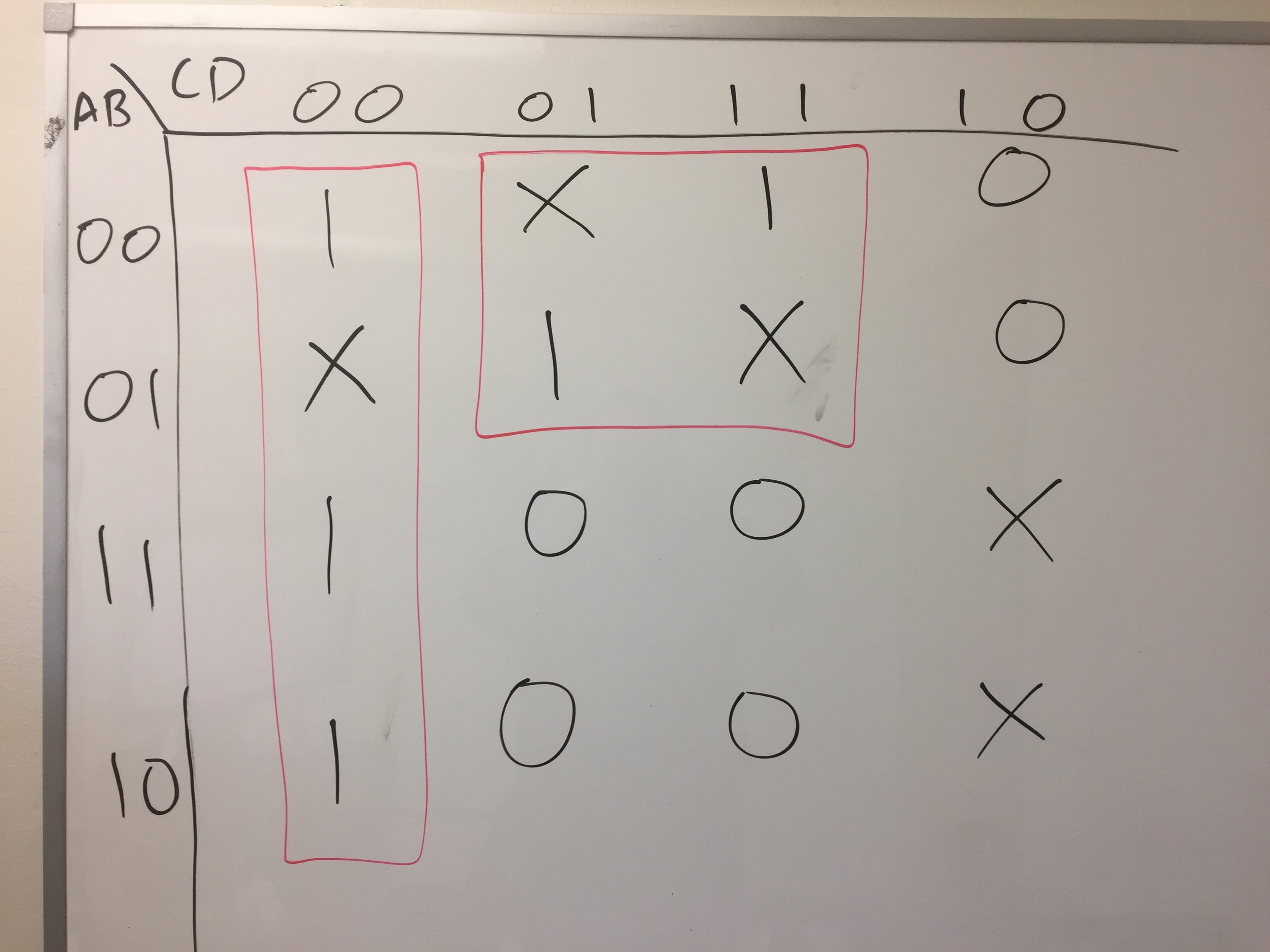3. An optimized sum-of-products equation, derived from the Karnaugh map created in the previous step.
```              U = !C!D + !AD
```
7. Design a two-bit arithmetic logic unit (ALU) that has the following operations:

• `AND` the two operands together
• `OR` the two operands together
• `NAND` the two operands together (that is, AND them and NOT the result)
• `NOR` the two operands together (that is, OR them and NOT the result)

Specifically, your ALU will have the following inputs:

Input Name Input Description
`A0` Bit 0 of the first operand
`A1` Bit 1 of the first operand
`B0` Bit 0 of the second operand
`B1` Bit 1 of the second operand
`S0` Select bit 0, used for specifying the operation to perform (see table below)
`S1` Select bit 1, used for specifying the operation to perform (see table below)

Given the above inputs, your ALU will produce the following outputs:

Output Name Output Description
`U0` Bit 0 of the output
`U1` Bit 1 of the output

As for which operation should be performed, this is based on the values of inputs `S0` and `S1`. The table below described the values that correspond to the different operations:

Value for `S1` Value for `S0` Operation
`0` `0` `AND`
`0` `1` `OR`
`1` `0` `NAND`
`1` `1` `NOR`

For this task, you may use the following provided components, in unlimited supply:

• AND, OR, and NOT gates
• 4-input multiplexers, which take the following:
• Two selector bits: `S1` and `S0`
• Four operands: `A`, `B`, `C`, and `D`
Given the above inputs, 4-input multiplexers produce a single output `Z`. They should be drawn using the symbol below: